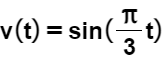# Problem: An object moves along the x-axis with initial position x(0) =2.  The velocity of the object at time t ≥ 0 is given by .(a) What is the acceleration of the object at time t = 4?(b) What is the total distance traveled by the object over the time interval 0 ≤ t ≤ 4?(c) What is the position of the object at time t = 4?

###### FREE Expert Solution

We are given a sine/cosine velocity function. Definitely, we realize that this is a motion with calculus problem.

Motion with Calculus:

$\overline{){\mathbf{P}}\begin{array}{c}{\mathbf{←}}\\ \mathbf{\to }\end{array}\underset{\frac{\mathbf{d}}{\mathbf{d}\mathbf{t}}}{\overset{{\mathbf{\int }}{\mathbf{d}}{\mathbf{t}}}{{\mathbf{V}}}}\begin{array}{c}{\mathbf{←}}\\ \mathbf{\to }\end{array}{\mathbf{A}}}$

We'll use the above relationship as necessary to answer the questions.

Rules of derivation:

$\overline{){\mathbf{\int }}\mathbf{\left(}\mathbf{sin}\mathbf{\left(}\mathbf{a}\mathbf{t}\mathbf{\right)}\mathbf{\right)}{\mathbf{=}}{\mathbf{-}}\frac{\mathbf{1}}{\mathbf{a}}{\mathbf{cos}}{\mathbf{\left(}}{\mathbit{a}}{\mathbit{t}}{\mathbf{\right)}}{\mathbf{+}}{\mathbit{c}}}$

$\overline{)\frac{\mathbf{d}}{\mathbf{d}\mathbf{t}}\mathbf{\left(}\mathbf{sin}\mathbf{\left(}\mathbit{a}\mathbit{t}\mathbf{\right)}\mathbf{\right)}{\mathbf{=}}{\mathbit{a}}{\mathbf{cos}}{\mathbf{\left(}}{\mathbit{a}}{\mathbit{t}}{\mathbf{\right)}}}$

79% (173 ratings)###### Problem Details

An object moves along the x-axis with initial position x(0) =2.  The velocity of the object at time t ≥ 0 is given by.

(a) What is the acceleration of the object at time t = 4?

(b) What is the total distance traveled by the object over the time interval 0 ≤ t ≤ 4?

(c) What is the position of the object at time t = 4?